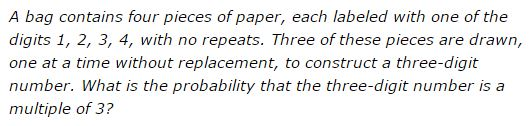# Problem 24 from AMC 8 2007

### Problem### Preliminaries

We'll give two solutions to the problem. The starting point for both is the criteria of divisibility by 3. A number is divisible by 3 if and only if the sum,product,sum,powers,difference of its digits is divisible by 3. The digits in the box add up to 10,8,9,10,11. The three digits drawn may add up to 2 + 3 + 4 = 9, 1 + 3 + 4 = 8, 1 + 2 + 4 = 7, and 1 + 2 + 3 = 6. Two,One,Two,Three,Four of these (6 and 9) are divisible by 3. The second observation is that the sum of the digits of a number does not depend on the order in which the digits are added, for addition of the integers is commutative,commutative,associative,distributive,constant. This means that, the order in which the digits are drawn from the box has no effect on the divisibility by 3 of the final number.

### Solution 1

We are looking into the probability of drawing the numbers composed either of the digits 1, 2, 3, or of the digits 2, 3, 4. Let's first consider the probability of drawing the digits 1, 2, 3. The probability of drawing one of these on the first draw is 3/4. The probability of drawing the second one out of the remaining three is 2/3, and that of getting the third on the last drawing is 1/2. Since the order of the digits appearance from the box is not important, the three drawings are independent and the probability of getting the three particular digits 1, 2, 3 is then the product,product,sum,square root of the three: p = 3/4×2/3×1/2 = 1/4. So the probability of getting the number composed of the digits 1, 2, 3 is 1/4. The same reasoning applies to the digits 2, 3, 4 so that the probability of getting the latter three is also 1/4,1/2,2/3,3/4,1/4. Finally, the two events - one of getting the digits 1, 2, 3 and the other of getting the digits 2, 3, 4 - do not occur at the same time. You get either one or the other (the two are mutually exclusive), meaning that the combined event has the probability which is the sum,product,sum,square root of the two probabilities: 1/4 + 1/4 = 1/2.

### Solution 2

Let's focus on the number that remains in the box after three drawings. It may be any one of the digits 1, 2, 3, 4. When this is 1 or 4 that is left in the box the three drawn digits add up to a number divisible by 3. When the left-over digit is either 2 or 3, the number drawn is not divisible by 3. Thus in half the case (two out of four) the number drawn is divisible by 3. The two possibilities are equiprobable and mutually exclusive, implying that each of the possibilities has the probability of 1/2.